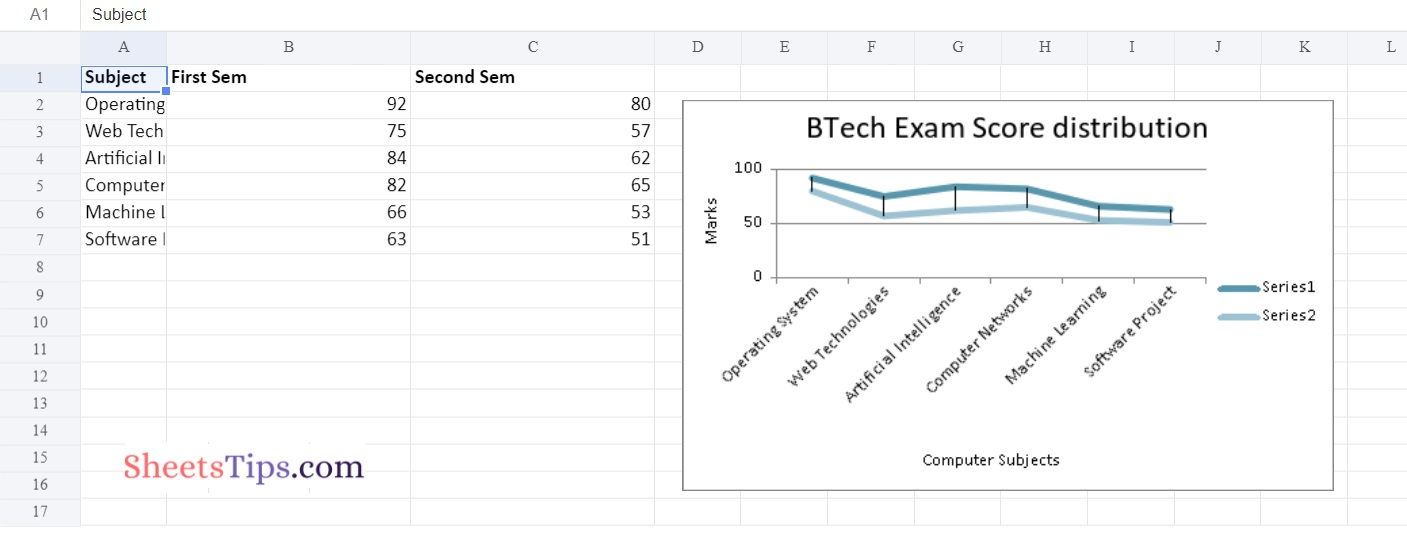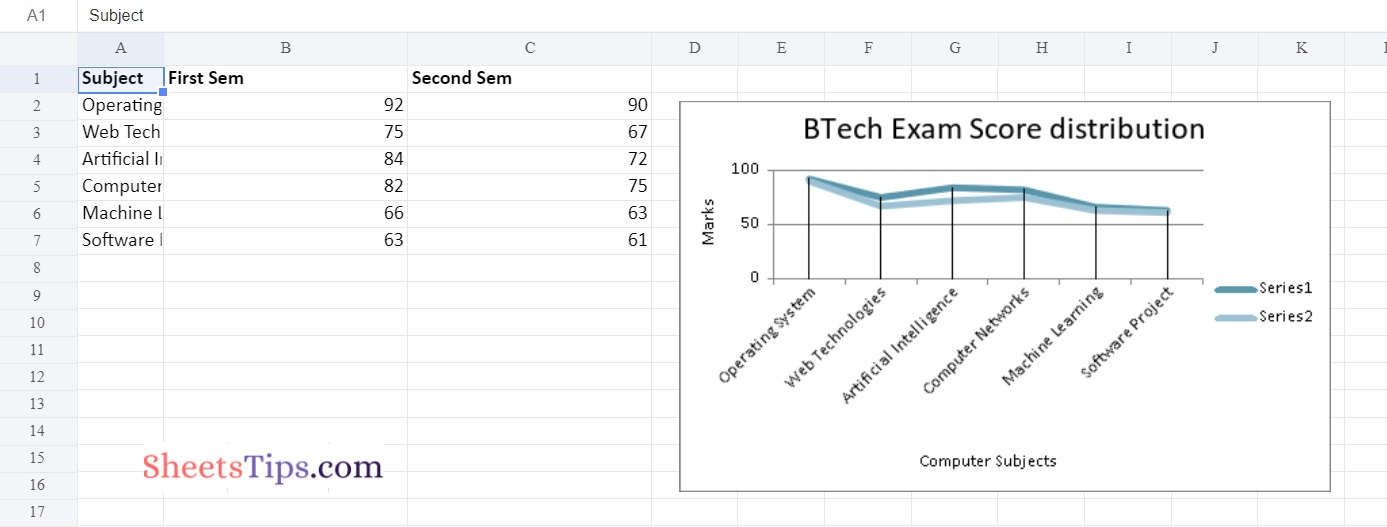# Python Program to Plot Charts in Excel Sheet with Data Tools using XlsxWriter module | Set – 1

in Excel Sheet with Data Tables using the XlsxWriter Module in Python.

Charts are made up of a series of one or more data points. The series are made up of references to cell ranges. To plot the charts on an excel sheet, first create a chart object of the desired chart type ( i.e Stock chart, etc.). After generating chart objects, insert data into them before adding them to the sheet object.

Install the xlsxwriter using the below code

`pip install xlsxwriter`

Looking in indexes: https://pypi.org/simple, https://us-python.pkg.dev/colab-wheels/public/simple/
Collecting xlsxwriter
|████████████████████████████████| 149 kB 5.0 MB/s
Installing collected packages: xlsxwriter
Successfully installed xlsxwriter-3.0.3

## Program to Plot Charts in Excel Sheet with Data Tools using XlsxWriter module in Python| Set – 1

### Chart #1: High-Low Lines Chart

Approach:

Approach:

• Import the xlsxwriter library using the import function.
• Pass the excel file path to the Workbook() function of xlsxwriter which is the file we want to create and store it in a variable.
• Create the new Worksheet using the add_worsheet() function and apply it to the above workbook object and store it in a variable.
• Create a new Format object to format cells in spreadsheets using the add_format() method and apply it to the above workbook object.
• Here, we created a bold format object.
• Add the worksheet data that the charts will use.
• Write a row of heading data starting with ‘A1’ in the bold format using the write_row() function and apply it to the above worksheet object by passing the argument row number, headings list, and format.
• Write the column data that is starting from ‘A2’, ‘B2’, and ‘C2’ using the write_coulmn() function and pass data as an argument to it.
• Write a column of data starting from ‘A2’, ‘B2’, and ‘C2’ respectively.
• Change the width of the column using the set_column() function.
• Using the add_chart() method, create a chart object that can be added to a worksheet.
• Pass the type of chart as an argument to the add_chart() function and apply it to the above workbook object.
• Using the add series() method, you can add a data series with a pattern to a chart.
• Set up the High-Low lines using the set_high_low_lines() function
• The gap is used to highlight the patterns.
• Configuring the second series.
• Set the title to the chartObject using the set_title() function by passing the title name as value and key as “name” (Here the arguments are object).
• Label the x axis using the set_x_axis() function by passing the Computer Subjects as value and key as “name” (Here the arguments are object).
• Label the y axis using the set_x_axis() function by passing the Marks as value and key as “name” (Here the arguments are object).
• Set the style of the chart using the set_style() function.
• Add a chartObject to the worksheet with the provided offset values in the top-left corner of a chartObject that is anchored to cell D2.
• Close the workbook Object using the close() function.
• The Exit of the Program.

Below is the Implementation:

```# Import the xlsxwriter library using the import function
import xlsxwriter

# Pass the excel file path to the Workbook() function of xlsxwriter
# which is the file we want to create and store it in a variable
workbookObj = xlsxwriter.Workbook('chartHighLow.xlsx')

# Create the new Worksheet using the add_worsheet() function and
# apply it to the above workbook object and store it in a variable

# Create a new Format object to format cells in spreadsheets using the add_format() method and apply it to the above workbook object.
# Here, we created a bold format object. .

# Add the worksheet data that the charts will use.
headingsData = ['Subject', 'First Sem', 'Second Sem']
worksheetData= [["Operating System", "Web Technologies", "Artificial Intelligence",
"Computer Networks", "Machine Learning", "Software Project Management"],
[92, 75, 84, 82, 66, 63],
[90, 67, 72, 75, 63, 61]]

# Write a row of heading data starting with 'A1' in bold format using the write_row() function
# and apply on the above worksheet object
# by passing the argument rowNumber,headings list,and format.

# Write the column data that is starting from 'A2','B2','C2'
# using the write_coulmn() function
# and pass data as argument to it
# Write a column of data starting from
# 'A2', 'B2', 'C2' respectively .
newWorksheet.write_column('A2', worksheetData)
newWorksheet.write_column('B2', worksheetData)
newWorksheet.write_column('C2', worksheetData)

# Change the width of the column using the set_column() function
newWorksheet.set_column('B:C', 25)

# Using the add_chart() method, create a chart object that can be added to a worksheet.
# Pass the type of chart as an argument to the add_chart() function and apply it to the above workbook object

# Set up the High-Low lines using the set_high_low_lines() function
chartObject.set_high_low_lines()

# Using the add series() method, you can add a data series with a pattern to a chart.
# The gap is used to highlight the patterns.

'categories': '= Sheet1 !\$A\$2:\$A\$7',
'values':     '= Sheet1 !\$B\$2:\$B\$7',
})

# Configuring the second series.
'categories': '= Sheet1 !\$A\$2:\$A\$7',
'values':     '= Sheet1 !\$C\$2:\$C\$7',
})

# Set the title to the chartObject using the set_title() function by passing the title name as value and key as "name" (Here the arguments are object)
chartObject.set_title({'name': 'BTech Exam Score distribution'})

# Label the x axis using the set_x_axis() function by passing the Computer Subjects as value and key as "name" (Here the arguments are object)
chartObject.set_x_axis({'name': 'Computer Subjects'})

# Label the y axis using the set_x_axis() function by passing the Marks as value and key as "name" (Here the arguments are object)
chartObject.set_y_axis({'name': 'Marks'})

# Set the style of the chart using the set_style() function
chartObject.set_style(15)

# Add a chartObject to the worksheet with the provided offset values in the top-left corner of a chartObject that is anchored to cell D2.
newWorksheet.insert_chart('D2', chartObject,
{'x_offset': 20, 'y_offset': 5})

# Close the workbook Object using the close() function.
workbookObj.close()```

Output:### Chart #2: Drop Lines Chart

Set the chart object’s set_drop_lines() method to plot this type of chart on an excel sheet.

Below is the Implementation:

```# Import the xlsxwriter library using the import function
import xlsxwriter

# Pass the excel file path to the Workbook() function of xlsxwriter
# which is the file we want to create and store it in a variable
workbookObj = xlsxwriter.Workbook('chartDropLines.xlsx')

# Create the new Worksheet using the add_worsheet() function and
# apply it to the above workbook object and store it in a variable

# Create a new Format object to format cells in spreadsheets using the add_format() method and apply it to the above workbook object.
# Here, we created a bold format object. .

# Add the worksheet data that the charts will use.
headingsData = ['Subject', 'First Sem', 'Second Sem']
worksheetData = [["Operating System", "Web Technologies", "Artificial Intelligence",
"Computer Networks", "Machine Learning", "Software Project Management"],
[92, 75, 84, 82, 66, 63],
[90, 67, 72, 75, 63, 61]]

# Write a row of heading data starting with 'A1' in bold format using the write_row() function
# and apply on the above worksheet object
# by passing the argument rowNumber,headings list,and format.

# Write the column data that is starting from 'A2','B2','C2'
# using the write_coulmn() function
# and pass data as argument to it
# Write a column of data starting from
# 'A2', 'B2', 'C2' respectively .
newWorksheet.write_column('A2', worksheetData)
newWorksheet.write_column('B2', worksheetData)
newWorksheet.write_column('C2', worksheetData)

# Change the width of the column using the set_column() function
newWorksheet.set_column('B:C', 25)

# Using the add_chart() method, create a chart object that can be added to a worksheet.
# Pass the type of chart as an argument to the add_chart() function and apply it to the above workbook object

#  Set up the Drop-lines using the set_drop_lines() function
chartObject.set_drop_lines()

# Using the add series() method, you can add a data series with a pattern to a chart.
# The gap is used to highlight the patterns.

'categories': '= Sheet1 !\$A\$2:\$A\$7',
'values':     '= Sheet1 !\$B\$2:\$B\$7',
})

# Configuring the second series.
'categories': '= Sheet1 !\$A\$2:\$A\$7',
'values':     '= Sheet1 !\$C\$2:\$C\$7',
})

# Set the title to the chartObject using the set_title() function by passing the title name as value and key as "name" (Here the arguments are object)
chartObject.set_title({'name': 'BTech Exam Score distribution'})

# Label the x axis using the set_x_axis() function by passing the Computer Subjects as value and key as "name" (Here the arguments are object)
chartObject.set_x_axis({'name': 'Computer Subjects'})

# Label the y axis using the set_x_axis() function by passing the Marks as value and key as "name" (Here the arguments are object)
chartObject.set_y_axis({'name': 'Marks'})

# Set the style of the chart using the set_style() function
chartObject.set_style(15)

# Add a chartObject to the worksheet with the provided offset values in the top-left corner of a chartObject that is anchored to cell D2.
newWorksheet.insert_chart('D2', chartObject,
{'x_offset': 20, 'y_offset': 5})

# Close the workbook Object using the close() function.
workbookObj.close()```

Output: